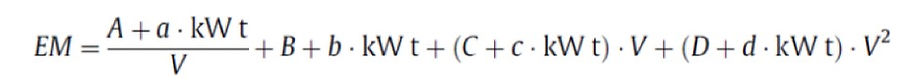top of pageThe purpose of this page is to allow you to create some of you own TNO results by entering in your own values for M and m or the empty and full vehicle mass. Using these new values you can see how the emissions vary based on the vehicle.

This basics of this page are the same as it uses the regression parameters for CO2, which are presented in Table 1, and it uses the two equations that are presented in the TNO model to first calculate the specific power of the vehicle, and then using this to calculate the emissions of CO2 per ton of weight and kilometre at the specified weight.

Then, in order to calculate your own TNO results, enter in the values that you want into the input box and press the calculate button to see how the emissions vary.

To get any clarification on the terms in this page, feel free to refer back to the TNO Model front page.

###### Model/Equation 1###### Equation 2###### Creating Our Results

Now that we have our equations and our regression parameters, the next step is to actually input our variables and to get our own TNO results.

The first thing you want to do is calculate the specific power. This is done in the first part of the calculator as you enter in the mass of the vehicle in tons, and the payload of the vehicle. Once you have done this, the specific power is automatically created.

Once you have the specific power of the vehicle, it is time to calculate the actual emissions of the vehicle. This is done by inputing in the velocity of the vehicle in kilometres per hour, and then hitting enter. Once you hit enter, the specific power will be calculated and implemented into the main equation to get the emissions per ton.

bottom of page